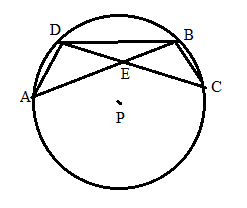Chapter 6.3, Problem 30EElementary Geometry For College St...

7th Edition
Alexander + 2 others
ISBN: 9781337614085

Solutions

Chapter
SectionElementary Geometry For College St...

7th Edition
Alexander + 2 others
ISBN: 9781337614085
Textbook Problem

In Exercises 27 to 30, provide a paragraph proof.Given: A B ¯ ≅ C D ¯     i n     ⊙ P Prove: Δ A B D ≅ Δ C D B .To determine

To find:

To prove ΔABDΔCDB.

Explanation

Given that, AB¯CD¯inP.

To prove ΔABDΔCDB

The diagrammatic representation is given below,

From the above figure shows that DAB,ABD,BCD,CDB have the vertex on the circle.

We know that inscribed angle is half of the arc measure. That is,

mDAB=mDB^2

mBCD=mDB^2

mDAB=mBC^2

Given that AB¯CD¯inP therefore, mADB^=mDBC^

Then add the arc to get the following,

Still sussing out bartleby?

Check out a sample textbook solution.

See a sample solution

The Solution to Your Study Problems

Bartleby provides explanations to thousands of textbook problems written by our experts, many with advanced degrees!

Get Started

Find the modes, medians, and means of the scores in Problems 17-22. 19. 14, 17, 20, 31, 17, 42

Mathematical Applications for the Management, Life, and Social Sciences

Convert the expressions in Exercises 6584 to power form. 4x23+x3/2623x2

Finite Mathematics and Applied Calculus (MindTap Course List)

Prove that limx21x=12.

Single Variable Calculus